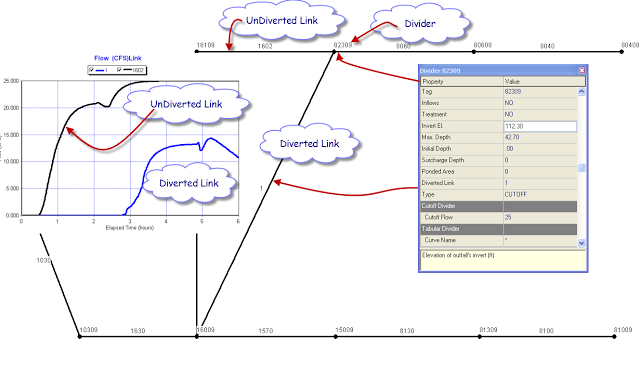# Cutoff Divider in the SWMM 5 Kinematic Wave Solution

Subject: Cutoff Divider in the SWMM 5 Kinematic Wave Solution

A divider node in the SWMM 5 Kinematic Wave solution will divide the inflow to a node for two downstream links based on three criteria:

1. Cutoff Divider,
2. Tabular Divider, and
3. Weir Divider

The rule for a Cutoff Divider is that the flow up to the Cutoff Flow will flow down the undiverted link and any flow over the cutoff flow will go down the diverted link. If the total inflow to a node the current flow in the undiverted link then the extra flow will go down the diverted link even though the flow in the undiverted link is not equal to the cutoff flow.Figure 1.  How the Cutoff Divider Works in SWMM 5

Translate »